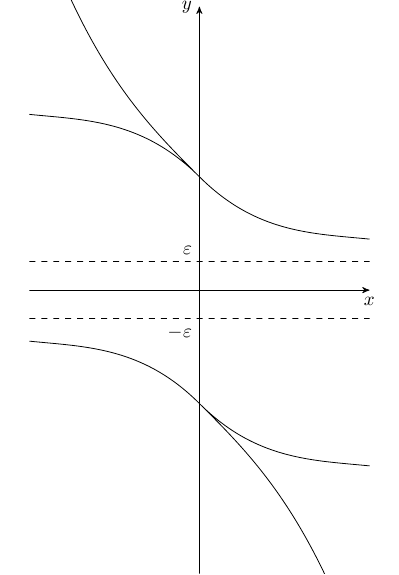# Find an f(x)

#### Alexmahone

##### Active member
Find an $\displaystyle f(x)$ such that $\displaystyle \frac{1}{f(x)}$ is defined for all $\displaystyle x$ and is bounded, but $\displaystyle f(x)$ is decreasing.

Last edited:

#### Evgeny.Makarov

##### Well-known member
MHB Math Scholar
This is not hard. Obviously, we must have f(x) ≠ 0 and moreover f(x) must be separated from 0, i.e., for some ε we must have |f(x)| > ε for all x.

#### Alexmahone

##### Active member
This is not hard. Obviously, we must have f(x) ≠ 0 and moreover f(x) must be separated from 0, i.e., for some ε we must have |f(x)| > ε for all x.
I'm still not able to find such a function.#### Amer

##### Active member
Find an $\displaystyle f(x)$ such that $\displaystyle \frac{1}{f(x)}$ is defined for all $\displaystyle x$ and is bounded, but $\displaystyle f(x)$ is decreasing.
what is the domain of the function ? all real numbers ?

#### Evgeny.Makarov

##### Well-known member
MHB Math Scholar
I'm still not able to find such a function.
You can't find a decreasing function whose graph lies outside the band $\{(x,y):|y|\le\varepsilon\}$? If you don't know a precise formula, can you at least describe how such function behaves?

#### Alexmahone

##### Active member
You can't find a decreasing function whose graph lies outside the band $\{(x,y):|y|\le\varepsilon\}$? If you don't know a precise formula, can you at least describe how such function behaves?
Since $\displaystyle f(x)$ is decreasing, $\displaystyle \frac{1}{f(x)}$ is increasing. But $\displaystyle \frac{1}{f(x)}$ is also bounded. So, it must approach a certain limit as $\displaystyle {x\to\infty}$.

#### Jester

##### Well-known member
MHB Math Helper
How about $f = 1 + e^{-x}$?

•Alexmahone

#### Evgeny.Makarov

##### Well-known member
MHB Math Scholar
Since $\displaystyle f(x)$ is decreasing, $\displaystyle \frac{1}{f(x)}$ is increasing. But $\displaystyle \frac{1}{f(x)}$ is also bounded. So, it must approach a certain limit as $\displaystyle {x\to\infty}$.
Yes, but I was asking really about f(x). Here are the possible behaviors of f(x).•Alexmahone